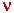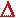Math & Science Home | Proficiency Tests | Mathematical Thinking in Physics | Aeronauts 2000

 CONTENTS Introduction Fermi's Piano Tuner Problem How Old is Old? If the Terrestrial Poles were to Melt... Sunlight Exerts Pressure Falling Eastward What if an Asteroid Hit the Earth Using a Jeep to Estimate the Energy in Gasoline How do Police Radars really work? How "Fast" is the Speed of Light? How Long is a Light Year? How Big is a Trillion? "Seeing" the Earth, Moon, and Sun to Scale Of Stars and Drops of Water If I Were to Build a Model of the Cosmos... A Number Trick Designing a High Altitude Balloon Pressure in the Vicinity of a Lunar Astronaut Space Suit due to Outgassing of Coolant Water Calendar Calculations Telling Time by the Stars - Sidereal Time Fields, an Heuristic Approach The Irrationality ofThe Irrationality ofThe Number (i)i Estimating the Temperature of a Flat Plate in Low Earth Orbit Proving that (p)1/n is Irrational when p is a Prime and n>1 The Transcendentality ofIdeal Gases under Constant Volume, Constant Pressure, Constant Temperature and Adiabatic Conditions Maxwell's Equations: The Vector and Scalar Potentials A Possible Scalar Term Describing Energy Density in the Gravitational Field A Proposed Relativistic, Thermodynamic Four-Vector Motivational Argument for the Expression-eix=cosx+isinx Another Motivational Argument for the Expression-eix=cosx+isinx Calculating the Energy from Sunlight over a 12 hour period Calculating the Energy from Sunlight over actual full day Perfect Numbers-A Case Study Gravitation Inside a Uniform Hollow Sphere Further note on Gravitation Inside a Uniform Hollow Sphere Pythagorean Triples Black Holes and Point Set Topology Additional Notes on Black Holes and Point Set Topology Field Equations and Equations of Motion (General Relativity) The observer in modern physics A Note on the Centrifugal and Coriolis Accelerations as Pseudo Accelerations - PDF File On Expansion of the Universe - PDF File

## Fields, an Heuristic Approach

Imagine a mathematical cube. Imagine also that the cube is empty. Now place a single mathematical point inside the cube. (The location of the point is arbitrary, so long as the point is inside.) Place a second point somewhere else inside the cube, and allow this second point to approach the first until the spacing between the two is arbitrarily small. Repeat this procedure over and over until the cube is densely filled with mathematical points; i.e., either (a) so that there is no volume inside the cube, regardless of how small, in which there is not at least one mathematical point, or, equivalently, (b) so that if, at any point P inside the cube, you were to construct a sphere with center at P, then allow the sphere to become arbitrarily small, there would always be at least one other point inside the sphere besides P. Call this space of mathematical points a continuum. In itself, the continuum has no other function than to serve as a substratum for constructing various types of mathematical spaces and fields. Let us now use this continuum of points to illustrate the concept of the field.

First, we must orient the cube. Choose an orientation such that you can distinguish a left face, a right face, and a face nearest you along whose normal line you are observing the cube. We shall use the left-right axis of the cube as a reference direction. Now, to every point inside the cube let us assign a color from the visible spectrum according to some agreed-upon rule. (Mathematically, this operation may be done by assigning a unique frequency to every point.) Call the color at any given point a field quantity, and call the resultant whole a color field (. . . so far, so good). A field is a continuum of points with a mathematical quantity assigned to every point according to some kind of rule. We will now consider three different types of color field, each constructed according to a different rule.

Time-Invariant (Static) Continuous Color Field: Imagine a color field in which the color changes 'gradually' from left to right, say starting with red at the left face of the cube and progressing smoothly through the rainbow to indigo at the right face. Let the colors remain non-varying with time. Such a field is called a time-invariant (static) continuous field. Now pick any two points along the left-right axis and compare their colors. There is a measurable difference (arithmetic difference in frequency). This difference becomes arbitrarily small as the points become arbitrarily close. When the distance between the points approaches zero, the ratio of the difference between the colors (frequencies) and the distance between the points approaches a finite value. This ratio is called the first derivative at the point, and, with x representing distance andrepresenting frequency, is written

d/dx = the limit, asx approaches zero, of the ratio/x

where the symbolis shorthand for "the difference in ..." The first derivative tells us how quickly the color changes with distance as we move along the left-right axis of the cube. (Actually, we were not limited to the left-right axis; any direction might have been chosen along which to evaluate the derivative; the left-right axis was merely chosen here for conceptual convenience).

If first derivatives are evaluated at two different points along the left-right axis and compared the same way, then it is also possible to show that the change in first derivative also becomes arbitrarily small as the points become arbitrarily close. Again, the ratio of the differences approaches a finite value. This ratio is called the second derivative, and is written

d 2/dx2.

The second derivative tells us how quickly the first derivative changes as we move along the left-right axis. Since the first and second derivatives are finite at every point inside the cube, they are said to exist everywhere within the field, and to be continuous functions of location. Such a field is said to be well behaved.

Discontinuous color field: Retain the previous orientation of the cube, 'erase' the continuous field (in your imagination), and divide the cube with a plane so that it is possible to speak of a right half cube and a left half cube. Now imagine a color field in which all the points in the left half cube are a uniform and vivid green, and all the points in the right half field are a uniform and vivid red. Each half field is well behaved, and, in fact, exhibits no change in color (frequency) from point to point whether the points are arbitrarily close or not (the first and second derivatives are all equal to zero). But conditions in the field near the plane dividing the two half cubes are very different: Consider one point PL in the left half cube, and a second point PR in the right half cube. The difference in the color (frequency) associated with these two points remains finite and constant, regardless of how arbitrarily close we allow the points to become. When the distance between the points approaches zero, the ratio of differences approaches infinity. Therefore, the derivatives along the plane separating the right and left half cubes do not exist (i.e., do not have finite values), and the field is said to be discontinuous everywhere along the plane. At such a field discontinuity, the field is not well behaved. (In some physical or engineering applications, such a discontinuity is called a shock boundary.)

Time-Varying Continuous Field: Now let us return to the continuous color field. We initially assumed that the colors remained constant with time; i.e., we assumed that the cube would appear always the same regardless of when we chose to look at it. We said that such a field was called a time invariant (static) field. But the assumption of time invariance is not essential to our example of a continuous field (or a discontinuous one for that matter). So now, beginning with the color field of example (1), imagine that, at every point in the field, the color is gradually (continuously) changing. In fact, let us specify a rule for the change to help the imagination along. First, imagine a line. Now, place the rainbow colors along the line with red at the left and indigo at the right. Place a movable pointer on the line. Now pick a point in the field, and place the pointer at the corresponding color on the line. Let the pointer gradually move to the right until it reaches the end of the line. Then let the pointer move to the left, and so on, back and forth. Do this same operation at every point in the field letting the pointer move at the same rate (speed) each time. The overall effect is that, everywhere in the field, the full spectrum will sooner or later be exhibited. If I stand at a single point, the color at that point will change gradually through the rainbow. If I stand outside the cube and observe the field as a whole, waves of color will appear to be traveling across the cube along the left-right axis. This field is of particular interest because most fields dealt with in physics or engineering exhibit wave phenomena; e.g., the transmission field of a radio or television station, the wave field on the surface of a lake of ocean. The field we have been describing is called a time varying (dynamic) field; and, since the overall color pattern repeats from time to time, the field is further called a periodic time varying field, with the period equal to the difference between any two successive times in which the cube appears the same. Points separated along a left-right axis will still exhibit different colors at any given time (as when a snapshot of the cube is taken), and this difference may be thought of in terms of a phase difference throughout the field. Thus, a point near the left face might be going from red to orange at the same time that a point near the right face is going from green to blue.

This heuristic model is offered to facilitate visualization of the field concept, a formidable concept that can be daunting when first encountered by students of mathematics or physics.

Please send suggestions/corrections to:
Web Related: David.Mazza@grc.nasa.gov
Technology Related: Joseph.C.Kolecki@grc.nasa.gov
Responsible NASA Official: Theresa.M.Scott (Acting)